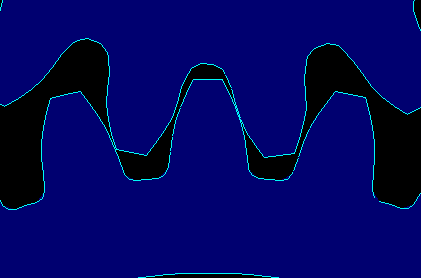Select Page

# NON LINEAR ANALYSIS

In a non-linear analysis, forces don’t display a linear relationship with deflections or stresses.

Optimum Solutions can analyze three major structural non-linearities:

• Geometric Non-linearity
• Material Non-linearity
• Contact (Boundaries Condition) Non-linearity

## Geometric Non-linearities:

It occurs in models that are subjected to severe deformations or strains. In these cases, the large deformation of the model has a sizable impact on the geometric characteristics of the model, which can cause the structure to respond non-linearly in stiffening and/or a softening manner. In general, geometric non-linearity must be taken into account when linear relationships cease to represent the actual deformation of the structure.

## Material Non-linearities:

Linear elasticity describes the material behavior only in a specific region of the stress-strain curve. All materials generally behave nonlinearly in some degree or at some time during their load history. Several factors can cause the material to behave in a nonlinear fashion. The dependency of the material stress-strain relation on the load history (as in plasticity problems), load duration (as in creep analysis), and temperature (as in thermo-plasticity) are some of these factors.

## Boundary Non-linearities:

In a linear analysis, it is assumed for small deformations, that superposition holds for the boundary conditions. As we know, this is not always the case due to the changing nature of the boundary conditions of structures involved in the analysis during motion, which means that the applied load will vary with the displacements of the structures. A typical example of Boundary non-linearities can be seen in gear-tooth contact analysis and follower forces.

The first question that you might ask yourself is why do I need to perform a nonlinear analysis?

The answer is simply; many physical phenomena that are observed cannot be described by a linear relationship. Linear analysis is only accurate for a model in which the relationship between the Forces and Stress/Displacements is linear in nature. In a nonlinear analysis, forces don’t display a linear relationship with deflections or stresses. Most structures behave nonlinear in one way or another, but in some specific cases a linear analysis may be adequate to represent the part’s behavior.

In all three cases the solution must be obtained by an incremental iterative method. The biggest problem in dealing with non-linear problems is the fact that a solution may not be found; in other words the iterative process can diverge. Furthermore the solution may not be unique and may not necessarily be the solution that is needed.

Optimum Solutions engineer staff use all the appropriate modeling techniques and solution algorithms, to obtained meaningful results in the appropriate time frame